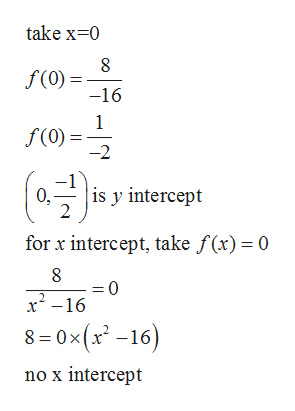# For the problem: f(x)=8/x^2-16a. Please find the x and y interceptsb. Test for symmetry: is the function even, odd, or neither?c. Find the vertical asymptotesThank you in advance!

Question

For the problem: f(x)=8/x^2-16

a. Please find the x and y intercepts

b. Test for symmetry: is the function even, odd, or neither?

c. Find the vertical asymptotes

check_circle

Step 1

Given function is

Step 2

For x and y intercepthelp_outlineImage Transcriptionclosetake x 0 8 f(0) = -16 1 f(0) -2 ( is y intercept 0, 2 for x intercept, take f(x)= 0 =0 x2-16 8 -0x(x2 -16) no x intercept fullscreen
Step 3

For even odd...

### Want to see the full answer?

See Solution

#### Want to see this answer and more?

Solutions are written by subject experts who are available 24/7. Questions are typically answered within 1 hour.*

See Solution
*Response times may vary by subject and question.
Tagged in

### Other